FanDuel 2019 NFC Championship Odds
+175
1.75 to 149ers
8.8% implied probability

+230
2.3 to 1Saints
7.3% implied probability

+600
6 to 1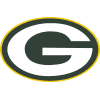Packers
3.4% implied probability

+600
6 to 1Seahawks
3.4% implied probability

+1100
11 to 1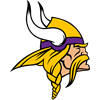Vikings
2.0% implied probability

+2500
25 to 1Cowboys
0.9% implied probability

+2500
25 to 1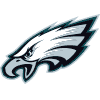Eagles
0.9% implied probability

+4000
40 to 1Rams
0.6% implied probability

+12000
120 to 1Bears
0.2% implied probability

+35000
350 to 1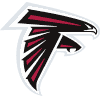Falcons
0.1% implied probability

+35000
350 to 1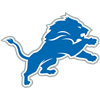Lions
0.1% implied probability

+100000
1000 to 1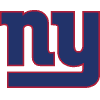Giants
0.0% implied probability

+100000
1000 to 1Cardinals
0.0% implied probability